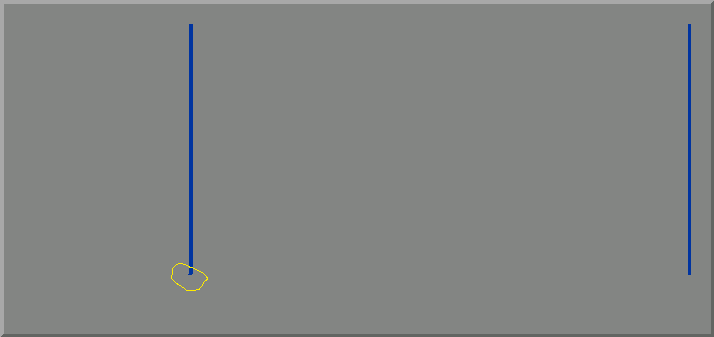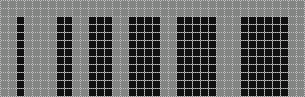{{ message }}
/ OpenTTD Public

# Drawing vertical/horizontal lines of width>1 problem#6865

Closed
opened this issue Jul 23, 2018 · 3 comments
Closed

# Drawing vertical/horizontal lines of width>1 problem#6865

opened this issue Jul 23, 2018 · 3 comments
Labels
bug needs triage stale

###J0anJosep commented Jul 23, 2018

 Blitter doesn't draw lines of width > 1 correctly: Sometimes there are some pixels moved at the beginning or end of the line. The width of the line doesn't match the one passed as an argument. `GfxDrawLine(x_zero, y_zero, x_zero, y_max, _colour_gradient[COLOUR_BLUE], 4);` `GfxDrawLine(x_max, y_zero, x_max, y_max, _colour_gradient[COLOUR_BLUE], 5);` See attached image:... where the left line should have a width of 5 and the right one a width of 4 pixels (and they have a width of 4 and 3 respectively). The text was updated successfully, but these errors were encountered:

###nielsmh commented Nov 4, 2018 • edited

 I can't reproduce the thickness issue. ``````GfxDrawLine(x_zero + 0, y_zero, x_zero + 0, y_max, 1, 1); GfxDrawLine(x_zero + 5, y_zero, x_zero + 5, y_max, 1, 2); GfxDrawLine(x_zero + 10, y_zero, x_zero + 10, y_max, 1, 3); GfxDrawLine(x_zero + 15, y_zero, x_zero + 15, y_max, 1, 4); GfxDrawLine(x_zero + 22, y_zero, x_zero + 22, y_max, 1, 5); GfxDrawLine(x_zero + 30, y_zero, x_zero + 30, y_max, 1, 6); ``````###andythenorth commented Jan 5, 2019

 So do we have a repro or not? :)

###stale bot commented Mar 25, 2019

 This issue has been automatically marked as stale because it has not had any activity in the last two months. If you believe the issue is still relevant, please test on the latest nightly and report back. It will be closed if no further activity occurs within 7 days. Thank you for your contributions.